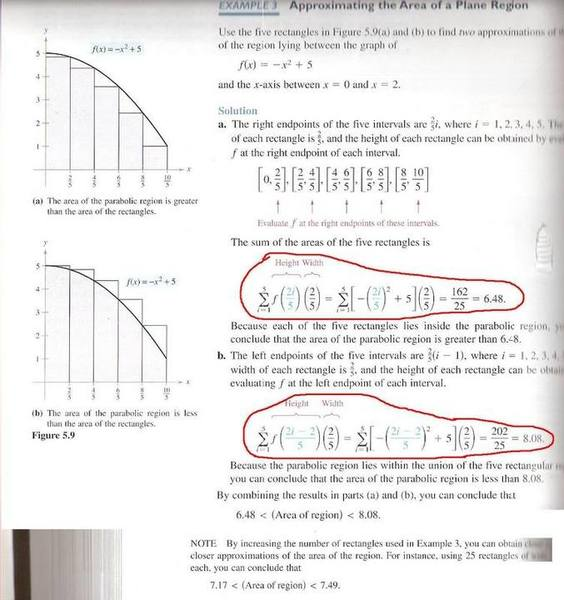Sigma notation

Homework StatementI'm just having trouble seeing how the book is getting from point-to-point. I understand the area=height X Width part of the equation, but I don't see how in the seconds step (2i/5)(2/5) turns into [-(2i/5)^2+5](2/5). Smae goes for the second step in the second equation circled in red.

$$f\left(\frac{2i}{5}\right)=-\left(\frac{2i}{5}\right)^2+5$$